# Test: Energy Stored in a Capacitor (NCERT)

## 15 Questions MCQ Test Topic-wise MCQ Tests for NEET | Test: Energy Stored in a Capacitor (NCERT)

Description
Attempt Test: Energy Stored in a Capacitor (NCERT) | 15 questions in 15 minutes | Mock test for NEET preparation | Free important questions MCQ to study Topic-wise MCQ Tests for NEET for NEET Exam | Download free PDF with solutions
QUESTION: 1

### A parallel plate capacitor is connected across a 2V battery and charged. The battery is then disconnected and a glass slab is introduced between plates. Which of the following pairs of quantities decreases?

Solution:

When battery is disconnected, charge remains constant. On introducing glass slab, capacity increases, Potential difference and energy stored decreases.

QUESTION: 2

### A capacitor has some dielectric between its plates, and the capacitor is connected to a dc source. The battery is now disconnected and then the dielectric is removed, then

Solution:

When the capacitor is connected to dc source, it gets charged. The battery is then disconnected, so no more charge can flow in. On removing dielectric capacitance decrease.
Energy stored (u = q2/2C) will increase.
Potential (V = q/C) will also increase.
Electric field (E = V/D) will increase.

QUESTION: 3

### A capacitor of capacitance 700 pF is charged by 100 V battery. The electrostatic energy stored by the capacitor is

Solution:

Here C = 700pF = 700 × 10−12F, V = 100V
Energy stored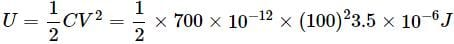QUESTION: 4

A 16 pF capacitor is connected to 70 V supply. The amount of electric energy stored in the capacitor is

Solution:

Here C = 16pF = 16 × 10−12FV = 80V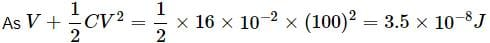QUESTION: 5

A capacitor is charged through a potential difference of 200 V, when 0.1 C charge is stored in it. The amount of energy released by it, when it is discharged is

Solution:

Here, V = 200V, q = 0.1C
Energy stored,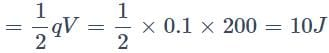When the capacitor is discharged, it releases the same amount of energy i.e., 10J

QUESTION: 6

A parallel plate capacitor has a uniform electric field E in the space between the plates. If the distance between the plates is d and area of each plate is A, the energy stored in the capacitor is

Solution:

Capacitance of a parallel plate capacitor is C = ε0A/d ………(i)
Potential difference between the plates is
V = Ed
The energy stored in the capacitor is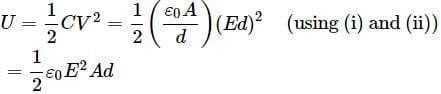QUESTION: 7

A metallic sphere of radius 18 cm has been given a charge of 5 × 10−6 C. The energy of the charged conductor is

Solution:

Here r = 18cm = 18 × 10−2m, q = 5 × 10−6C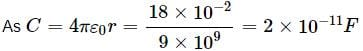Energy of charged conductor is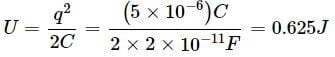QUESTION: 8

Two spherical conductors each of capacity C are charged to potential V and − V. These are then connected by means of a fine wire. The loss of energy will be

Solution:

Here, loss of energy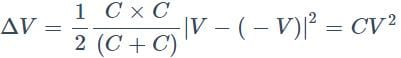QUESTION: 9

Two condensers, one of capacity C and other of capacity C/2 are connected to a V-volt battery, as shown in the figure. The work done in charging fully both the condensers isSolution:

As the capacitors are connected in parallel, therefore potential difference across both the condensors remains the same.Work done in charging fully both the condensors is given byQUESTION: 10

A parallel plate condenser with a dielectric of dielectric constant K between the plates has a capacity C and is charged to a potential V volt. The dielectric slab is slowly removed from between the plates and then reinserted. The net work done by the system in this process is

Solution:

The potential energy of a charged capacitor before removing the dielectric slat is U = Q2/2C.
The potential energy of the capacitor when the dielectric slat is first removed and the reinserted in the gap between the plates is U = Q2/2C
There is no change in potential energy, therefore work done is zero.

QUESTION: 11

Two identical capacitors have the same capacitance C. One of them is charged to potential V1 and the other to V2. The negative ends of the capacitors are connected together. When the positive ends are also connected, the decrease in energy of the combined system is

Solution:

Initial energy of the combined system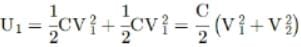On joining the two condensers in parallel, common potential.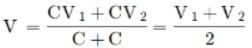∴ Final energy of the combined system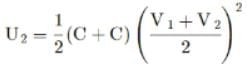Decrease in energy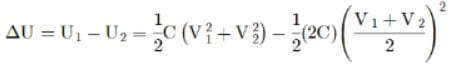QUESTION: 12

Energy stored in a capacitor and dissipated during charging a capacitor bear a ratio.

Solution:

Half of the energy is dissipated during charging a capacitor.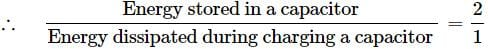QUESTION: 13

Two capacitors, 3μF and 4μF, are individually charged across a 6V6V battery. After being disconnected from the battery, they are connected together with the negative plate of one attached to the positive plate of the other. What is the final total energy stored

Solution: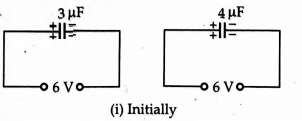As Q = CV
∴ Initially the charge on each capacitor is
Q1 = C1V1 = (3μF)(6V) = 18μC
and Q2 = C2V2 = (4μF)(6V) = 24μC
When two capacitors is are joined to each other such that negative plate of one is attached with the positive plate of the other. The charges Q1 and Q2 are redistributed till they attain the common potential which is given by.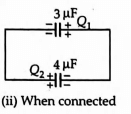Common potential V = Total Charge/Total capacitance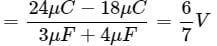Final energy stored,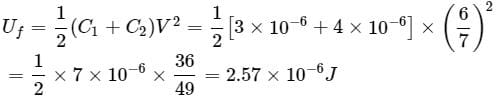QUESTION: 14

A parallel plate capacitor without any dielectric within its plates, has a capacitance C, and is connected to a battery of emf V. The battery is disconnected and the plates of the capacitor are pulled apart until the separation between the plates is doubled. What is the work done by the agent pulling the plates apart, in this process?

Solution:

Capacitance of a parallel plate capacitor is C = ε0A/d ……(i)
where A is the area of each plate and d is the distance between the plates. Initial energy stored in the capacitor,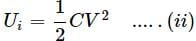When the separation between the plates is doubled , its capcaitance becomes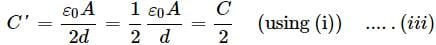As the bettary is disconnected , so charged capacitor becomes isolated and charge on it will reamain constant ,
∴ Q = Q
C'V = C'V (AsQ = CV)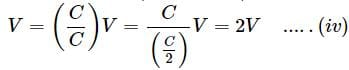Final energy stored in the capacitor ,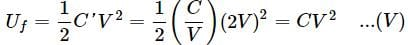Required work done,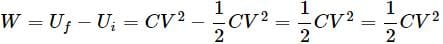QUESTION: 15

A series combination of n1, capacitors, each of value C1, is charged by a source of potential difference 4V. When another parallel combination of n2, capacitors, each of value C2, is charged by a source of potential difference V, it has the same (total) energy stored in it, as the first combination has. The value of C2, in terms of C1 is then

Solution: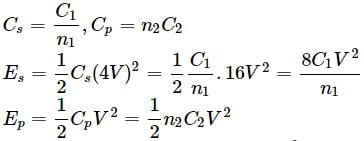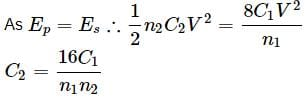Use Code STAYHOME200 and get INR 200 additional OFF Use Coupon Code